# How to use the AVERAGE function in Excel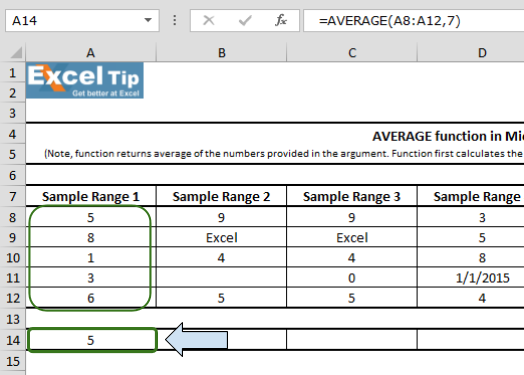In this article, we will learn How to use the AVERAGE function in Excel.

What is AVERAGE or MEAN? And What type of Excel functions use it?

AVERAGEA function is a built-in function to calculate the average or mean of the range of data values provided. The average or mean for the set of values is calculated using the formula shown below.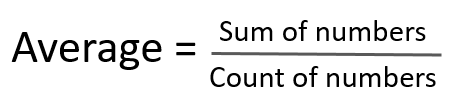Here

1. Sum of numbers is the mathematical addition of values.
2. Count of numbers (n) is the total count of values considered.

There are newer and updated versions of AVERAGE function used for a different set of dataset values.

1. AVERAGEA function : This function is used for values considering text as 0, TRUE as 1 and FALSE as 0 and numbers in text format.
2. AVERAGE function : This function is used for values considering only numbers which are in number format.
3. AVERAGEIF function : This function takes the average of values in range applying any one condition over the corresponding or the same range.
4. AVERAGEIFS function : This function takes the average of values in range applying any multiple condition over the corresponding or the same range.

AVERAGE Function in Excel

AVERAGE function calculates the average of the input array numbers ignoring text and logic values.

AVERAGE function Syntax:

 =AVERAGE(array)

array : input multiple number as array reference with the function. Like values at array A5:A500.

Example :

All of these might be confusing to understand. Let's understand how to use the function using an example.

Refer to these 7 different examples for the usage of Average function in Microsoft Excel on the basis of following sample data:

1st Example:

In the sample 1 we have few numbers for which we want to calculate average. Follow the steps given below:

• Enter AVERAGE function in cell A14
• =AVERAGE(A8:A12,7)
• Press Enter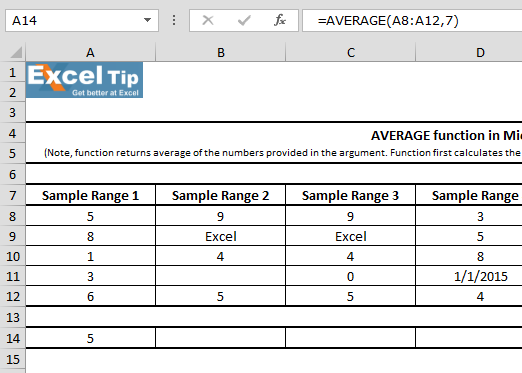Note: It can take up to 255 number of arguments in Excel 2007 or later versions.

Formula Explanation: A8 to A12 as range in the first argument, then we close the parentheses and hit enter. The function returns 4.6 as the average of these 5 numbers. Also, if you sum up these numbers 5 + 8 + 1 + 3 + 6 it will be equal to 23. And, as we have 5 numbers of cells in the argument, and when we divide 23 by 5, it returns 4.6 which is an average.

2nd Example:

In this example, we’ll see how AVERAGE function handles any empty cell or cells that contain text in the range, follow the steps given below:

• Enter AVERAGE function in cell B14
• Then, select B8:B12 as range
• =AVERAGE(B8:B12)
• Press Enter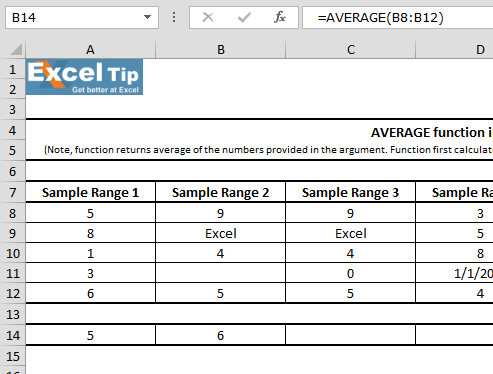Formula Explanation: In this case function returns 6 as average. Because cell B9 is ignored by AVERAGE function, since it contains text instead of a number and function found no value in B11.

3rd Example:

In this example, we’ll learn if there is a cell that contains zero in the range. Follow the steps given below:

• Enter function in cell C14
• Select C8 to C12 range
• =AVERAGE(C8:C12)
• Press Enter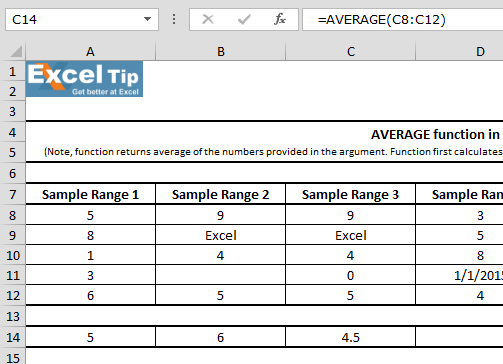4th Example:

In this example, we can see that one of the cell contains date within a range. So, we’ll learn that how Average function will work in case of any cell that contains date.

Follow given below steps:

• Enter the function in cell D14
• =AVERAGE(D8:D12)
• Press Enter

Function returns 8405. It is because in Excel, dates are saved as numbers. In this example, we have taken 1st Jan,2015 which is equivalent to 42005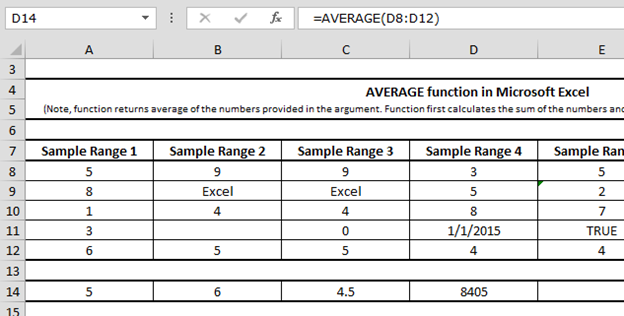5th Example:

In this example, we have taken text in the range for which we want to calculate average.

Follow the steps given below:

• Enter the function in cell E14
• =AVERAGE(E8:E12)
• Press Enter
• Function returns 4.5 as result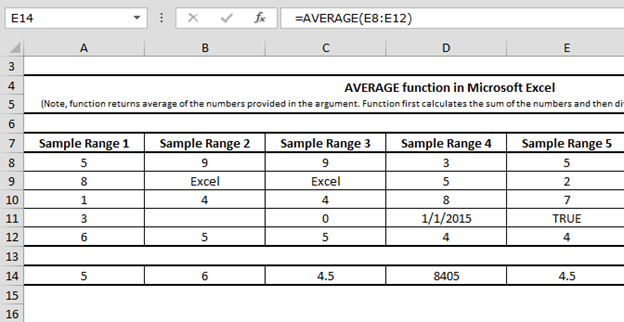It means Average function does not consider text value while performing the calculation. And by doing so it provides accurate results.

6th Example:

In this example, we’ll learn if a cell is having #N/A error within a range for which we want to calculate average, then how Average function will perform.

Follow the steps given below:

• Enter the function in cell F14
• =AVERAGE(F8:F12)
• Press Enter
• Function will return #N/A as resultIf any cell contains an error, the formula would also return error.

7th Example:

In this example, we’ll check if the selected range for average function is blank and how Average function will work.

Follow the steps given below:

• Enter the function in cell G14
• =AVERAGE(G8:G12)
• Press Enter

The function will return #DIV/0! Error because function did not find any value to be averaged in the argument.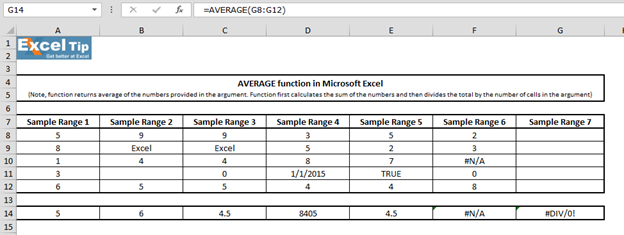In this way we can make use of the average function in MS-Excel.

Here are all the observational notes using the AVERAGE function in Excel
Notes :

1. The function returns #VALUE! criteria if no value matches the given criteria.

Video: How to use AVERAGE function in Excel

Watch the steps in this short video, and the written instructions are above the video

Hope this article about How to use the AVERAGE function in Excel is explanatory. You can use these functions in Excel 2016, 2013 and 2010. Find more articles on Mathematical operation and formulation with different criteria.

If you liked our blogs, share it with your friends on Facebook. And also you can follow us on Twitter and Facebook. We would love to hear from you, do let us know how we can improve, complement or innovate our work and make it better for you. Write us at info@exceltip.com

Related Articles :

How to use the AVERAGEIF function in Excel : This function takes the average of values in range applying any one condition over the corresponding or the same range.

How to use the AVERAGEIFS function in Excel : This function takes the average of values in range applying any multiple conditions over the corresponding or the same range.

How To Highlight Cells Above and Below Average Value : highlight values which are above or below the average value using the conditional formatting in Excel.

Ignore zero in the Average of numbers : calculate the average of numbers in the array ignoring zeros using AVERAGEIF function in Excel.

Calculate Weighted Average : find the average of values having different weight using SUMPRODUCT function in Excel.

Average Difference between lists : calculate the difference in average of two different lists. Learn more about how to calculate average using basic mathematical average formula.

Average numbers if not blank in Excel : extract average of values if cell is not blank in excel.

AVERAGE of top 3 scores in a list in excel : Find the average of numbers with criteria as highest 3 numbers from the list in Excel

Popular Articles :

How to use the IF Function in Excel : The IF statement in Excel checks the condition and returns a specific value if the condition is TRUE or returns another specific value if FALSE.

How to use the VLOOKUP Function in Excel : This is one of the most used and popular functions of excel that is used to lookup value from different ranges and sheets.

How to use the SUMIF Function in Excel : This is another dashboard essential function. This helps you sum up values on specific conditions.

How to use the COUNTIF Function in Excel : Count values with conditions using this amazing function. You don't need to filter your data to count specific values. Countif function is essential to prepare your dashboard.

## Comments

1.Hi! can you explain your requirement in detail.

Terms and Conditions of use

The applications/code on this site are distributed as is and without warranties or liability. In no event shall the owner of the copyrights, or the authors of the applications/code be liable for any loss of profit, any problems or any damage resulting from the use or evaluation of the applications/code.